# Classification from scratch, penalized Lasso logistic 5/8

June 4, 2018
By

Want to share your content on R-bloggers? click here if you have a blog, or here if you don't.

Fifth post of our series on classification from scratch, following the previous post on penalization using the $\ell_2$ norm (so-called Ridge regression), this time, we will discuss penalization based on the $\ell_1$ norm (the so-called Lasso regression).

First of all, one should admit that if the name stands for least absolute shrinkage and selection operator, that’s actually a very cool name… Funny story, a few years before, Leo Breiman introduce a concept of garrote technique… “The garrote eliminates some variables, shrinks others, and is relatively stable”.I guess that somehow, the lasso is the extension of the garotte technique

## Normalization of the covariates

As previously, the first step will be to consider linear transformations of all covariates $x_j$ to get centered and scaled variables (with unit variance)

 1 2 3 4  y = myocarde$PRONO X = myocarde[,1:7] for(j in 1:7) X[,j] = (X[,j]-mean(X[,j]))/sd(X[,j]) X = as.matrix(X) ## Ridge Regression (from scratch) The heuristics about Lasso regression is the following graph. In the background, we can visualize the (two-dimensional) log-likelihood of the logistic regression, and the blue square is the constraint we have, if we rewite the optimization problem as a contrained optimization problem,  1 2 3 4 5 6 7 8 9 10  LogLik = function(bbeta){ b0=bbeta beta=bbeta[-1] sum(-y*log(1 + exp(-(b0+X%*%beta))) - (1-y)*log(1 + exp(b0+X%*%beta)))} u = seq(-4,4,length=251) v = outer(u,u,function(x,y) LogLik(c(1,x,y))) image(u,u,v,col=rev(heat.colors(25))) contour(u,u,v,add=TRUE) polygon(c(-1,0,1,0),c(0,1,0,-1),border="blue")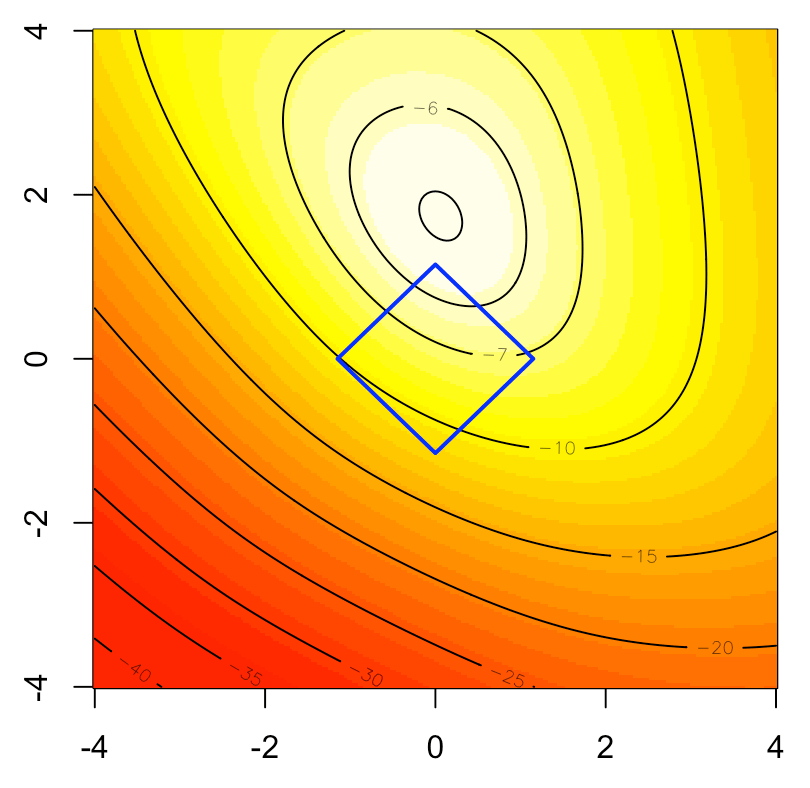The nice thing here is that is works as a variable selection tool, since some components can be null here. That’s the idea behind the following (popular) graph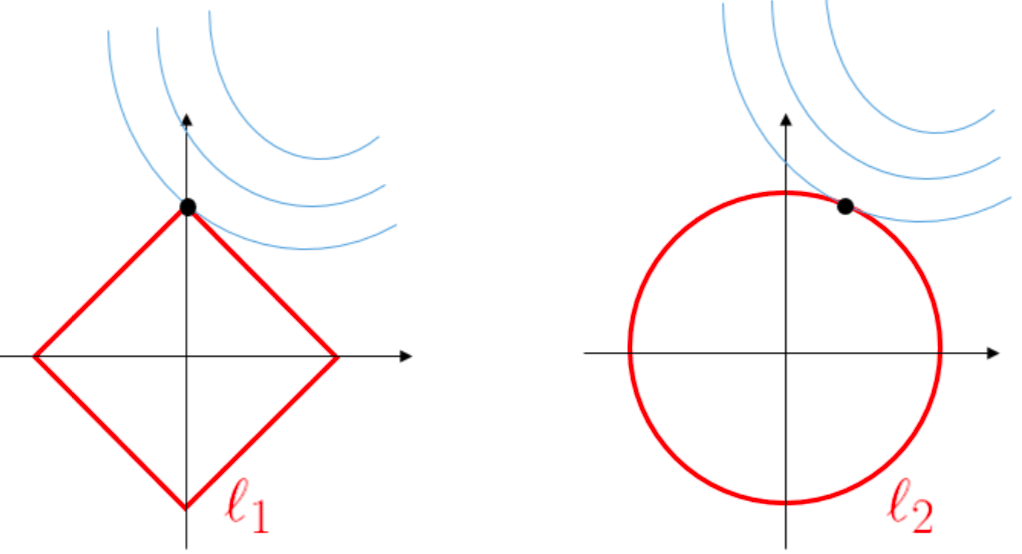(with lasso on the left, and ridge on the right). Heuristically, the maths explanation is the following. Consider a simple regression $y_i=x_i\beta+\varepsilon$, with $\ell_1$-penality and a $\ell_2$-loss fuction. The optimization problem becomes$$\min\big\{\mathbf{y}^T\mathbf{y}-2\mathbf{y}^T\mathbf{x}\beta+\beta\mathbf{x}^T\mathbf{x}\beta+2\lambda{\color{red}{|}}\beta{\color{red}{|}}\big\}$$The first order condition can be written$$-2\mathbf{y}^T\mathbf{x}+2\mathbf{x}^T\mathbf{x}\widehat{\beta}{\color{red}{\pm} }2\lambda=0$$(the sign in ${\color{red}{\pm}}$ being the sign of $\widehat{\beta}$). Assume that $\mathbf{y}^T\mathbf{x}>0$, then solution is $$\widehat{\beta}_{\lambda}^{lasso}=\max\left\lbrace\frac{\mathbf{y}^T\mathbf{x}-\lambda}{\mathbf{x}^T\mathbf{x}},0\right\rbrace$$(we get a corner solution when $\lambda$ is large). ## Optimization routine As in our previous post, let us start with standard (R) optimization routines, such as BFGS  1 2 3 4 5 6 7 8 9 10 11 12 13 14 15 16 17 18  PennegLogLik = function(bbeta,lambda=0){ b0=bbeta beta=bbeta[-1] -sum(-y*log(1 + exp(-(b0+X%*%beta))) - (1-y)*log(1 + exp(b0+X%*%beta)))+lambda*sum(abs(beta)) } opt_lasso = function(lambda){ beta_init = lm(PRONO~.,data=myocarde)$coefficients logistic_opt = optim(par = beta_init*0, function(x) PennegLogLik(x,lambda), hessian=TRUE, method = "BFGS", control=list(abstol=1e-9)) logistic_opt$par[-1] } v_lambda=c(exp(seq(-4,2,length=61))) est_lasso=Vectorize(opt_lasso)(v_lambda) library("RColorBrewer") colrs=brewer.pal(7,"Set1") plot(v_lambda,est_lasso[1,],col=colrs,type="l") for(i in 2:7) lines(v_lambda,est_lasso[i,],col=colrs[i],lwd=2)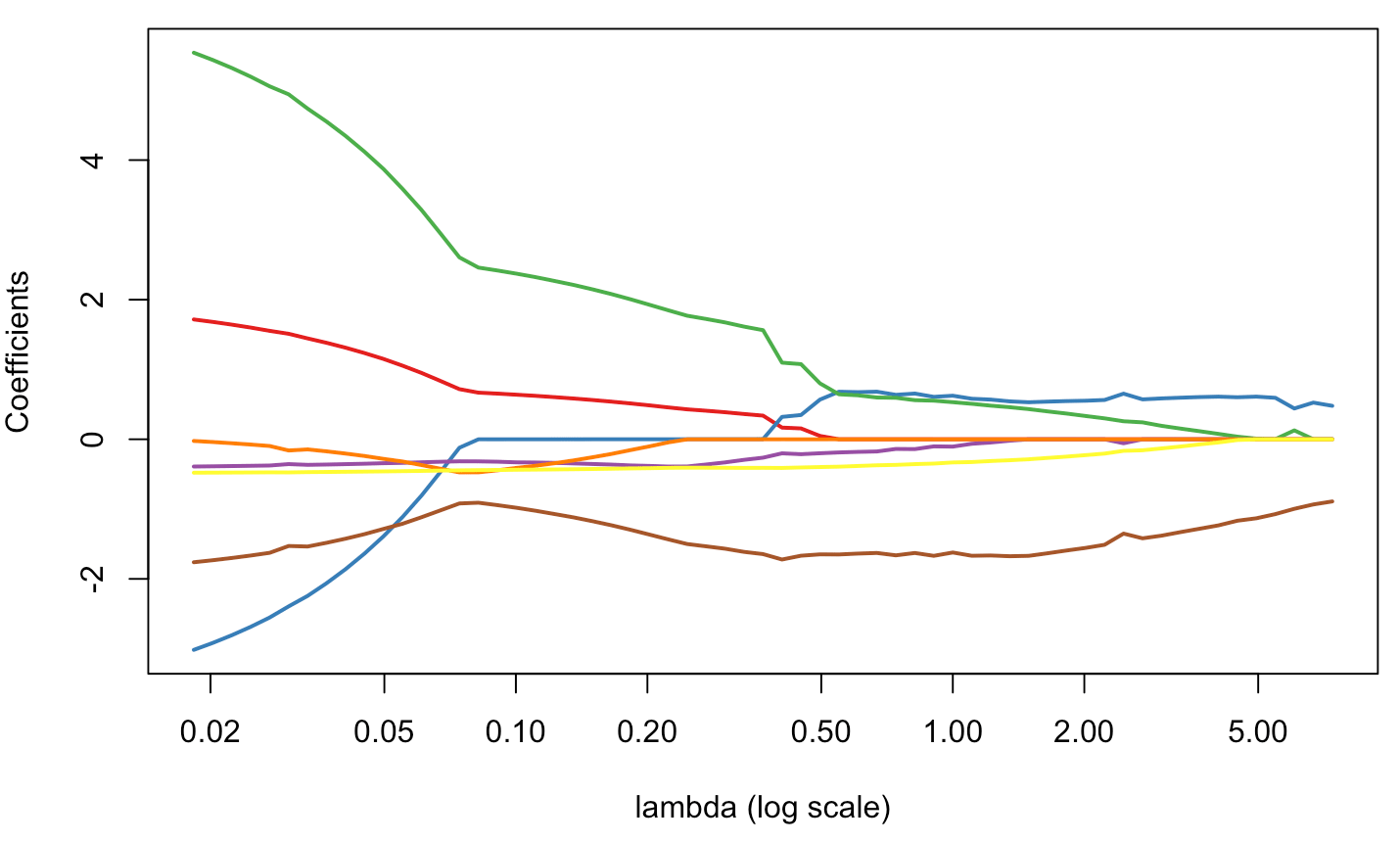But it is very heratic… or non stable. ## Using glmnet Just to compare, with R routines dedicated to lasso, we get the following  1 2 3  library(glmnet) glm_lasso = glmnet(X, y, alpha=1) plot(glm_lasso,xvar="lambda",col=colrs,lwd=2)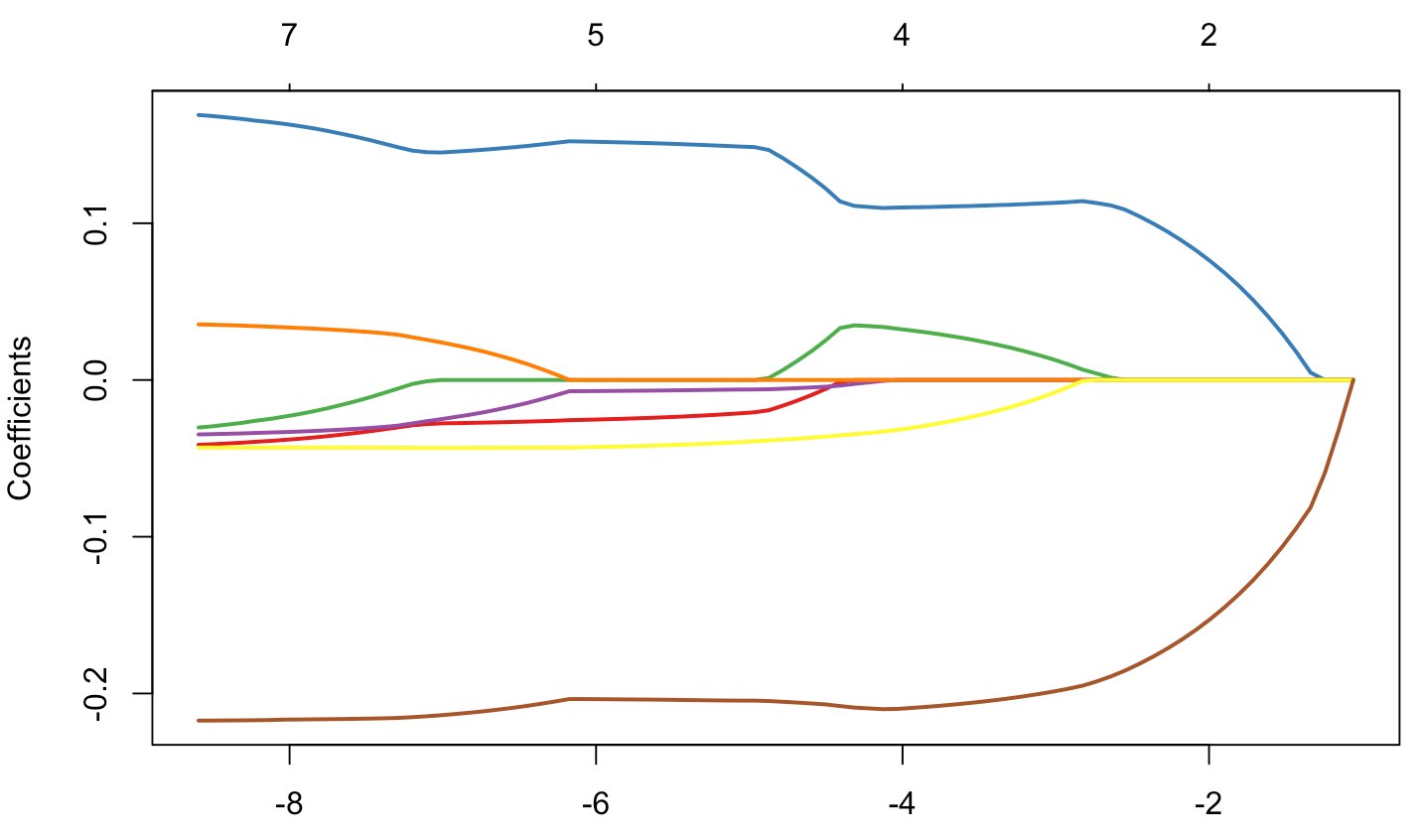1  plot(glm_lasso,col=colrs,lwd=2)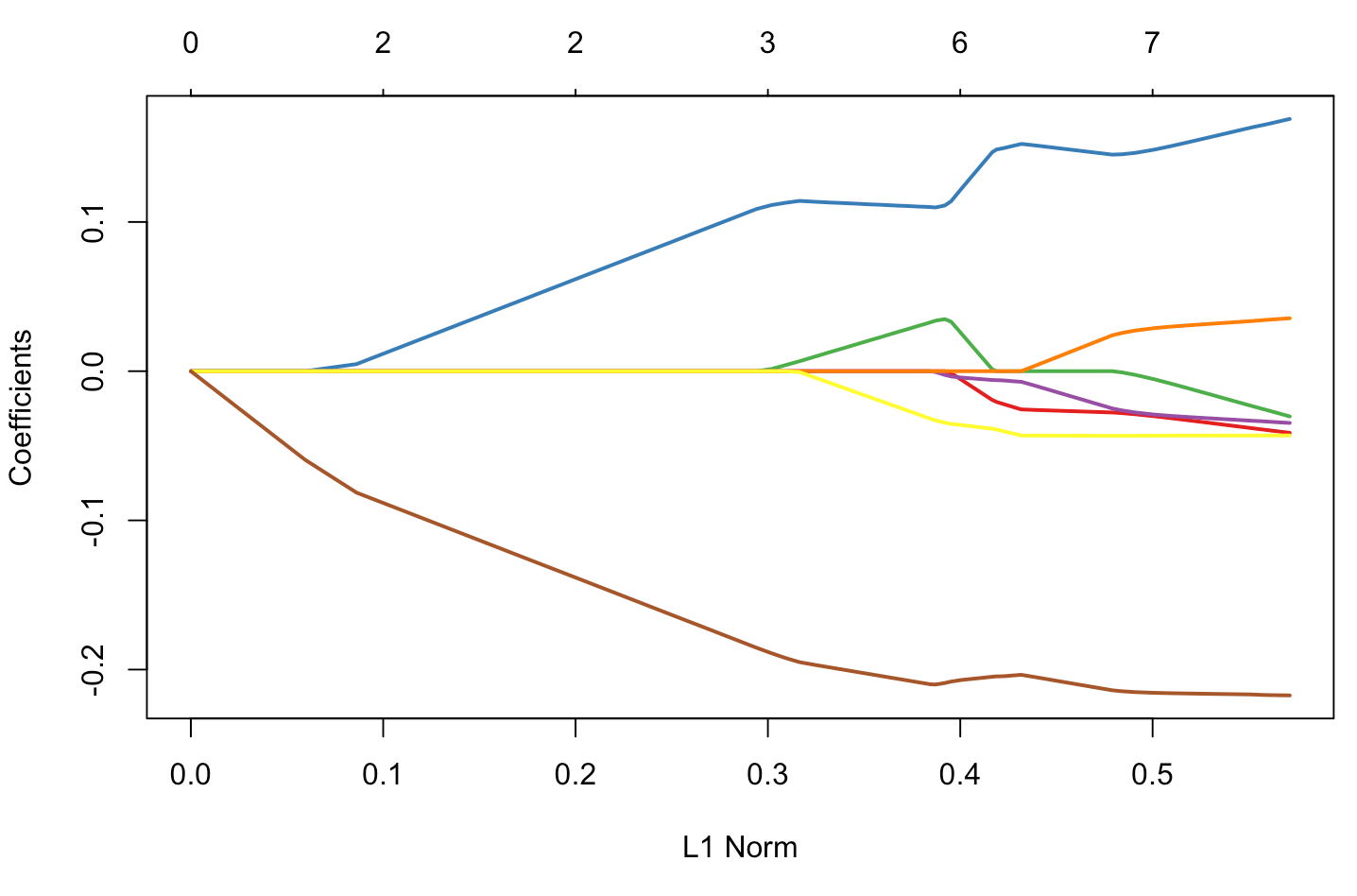If we look carefully what’s in the ouput, we can see that there is variable selection, in the sense that some $\widehat{\beta}_{j,\lambda}=0$, in the sense “really null”  1 2 3 4 5 6 7 8 9 10  glmnet(X, y, alpha=1,lambda=exp(-4))$beta 7x1 sparse Matrix of class "dgCMatrix" s0 FRCAR . INCAR 0.11005070 INSYS 0.03231929 PRDIA . PAPUL . PVENT -0.03138089 REPUL -0.20962611

Of course, with out optimization routine, we cannot expect to have null values

 1 2 3 4 5  opt_lasso(.2) FRCAR INCAR INSYS PRDIA 0.4810999782 0.0002813658 1.9117847987 -0.3873926427 PAPUL PVENT REPUL -0.0863050787 -0.4144139379 -1.3849264055

So clearly, it will be necessary to spend more time today, to understand how it works…

## Orthogonal covariates

Before getting into the maths, observe that when covariates are orthogonal, there is some very clear “variable” selection process,

 1 2 3 4 5 6  library(factoextra) pca = princomp(X) pca_X = get_pca_ind(pca)$coord glm_lasso = glmnet(pca_X, y, alpha=1) plot(glm_lasso,xvar="lambda",col=colrs) plot(glm_lasso,col=colrs)## Interior Point approach The penalty is now expressed using the $\ell_1$ so intuitively, it should be possible to consider algorithms related to linear programming. That was actually suggested in Koh, Kim & Boyd (2007), with some implementation in matlab, see http://web.stanford.edu/~boyd/l1_logreg/. If I can find some time, later one, maybe I will try to recode it. But actually, it is not the technique used in most R functions. Now, o be honest, we face a double challenge today: the first one is to understand how lasso works for the “standard” (least square) problem, the second one is to see how to adapt it to the logistic case. ## Standard lasso (with weights) If we get back to the original Lasso approach, the goal was to solve$$\min\left\lbrace\frac{1}{2n}\sum_{i=1}^n [y_i-(\beta_0+\mathbf{x}_i^T\mathbf{\beta})]^2+\lambda \sum_j |\beta_j|\right\rbrace$$(with standard notions, as in wikipedia or Jocelyn Chi’s post – most of the code in this section is inspired by Jocelyn’s great post). Observe that the intercept is not subject to the penalty. The first order condition is then$$\frac{\partial}{\partial\beta_0}\|\mathbf{y}-\mathbf{X}\mathbf{\beta}-\beta_0\mathbf{1}\|^2=(\mathbf{X}\mathbf{\beta}-\mathbf{y})^T\mathbf{1}+\beta_0\|\mathbf{1}\|^2=0$$i.e.$$\beta_0=\frac{1}{n^2}(\mathbf{X}\mathbf{\beta}-\mathbf{y})^T\mathbf{1}$$Assume now that KKT conditions are satisfied, since we cannot differentiate (to find points where the gradient is $\mathbf{0}$), we can check if $\mathbf{0}$ contains the subdifferential at the minimum.Namely$$\mathbf{0}\in\partial \left(\frac{1}{2}\|\mathbf{y}-\mathbf{X}\mathbf{\beta}\|^2+\lambda\|\mathbf{\beta}\|_{\ell_1}\right)=\frac{1}{2}\nabla\|\mathbf{y}-\mathbf{X}\mathbf{\beta}\|^2+\partial(\lambda\|\mathbf{\beta}\|_{\ell_1})$$ For the term on the left, we recognize $$\frac{1}{2}\nabla\|\mathbf{y}-\mathbf{X}\mathbf{\beta}\|^2=-\mathbf{X}^T(\mathbf{y}-\mathbf{X}\mathbf{\beta})=-\mathbf{g}$$so that the previous equation can be writen$$g_k\in\partial(\lambda|\beta_k|)=\begin{cases}\{+\lambda\}\text{ if }\beta_k>0 \\ \{-\lambda\}\text{ if }\beta_k<0 \\ (-\lambda,+\lambda)\text{ if }\beta_k=0\end{cases}[/latex]i.e. if $\beta_k\neq 0$, then $g_k = \text{sign}(\beta_k)\cdot\lambda$. Then we write the KKT conditions for this formulation and simplify them to produce a set of rules for checking our solution We can split $\beta_j$$ into a sum of its positive and negative parts by replacing $\beta_j$ with $\beta_j^+-\beta_j^-$ where $\beta_j^+,\beta_j^-\geq0$. Then the Lasso problem becomes$$-\log\mathcal{L}(\mathbf{\beta})+\lambda\sum_j(\beta_j^+-\beta_j^-)$$with constraints $\beta_j^+-\beta_j^-$. Let $\alpha_j^+,\alpha_j^-$ denote the Lagrange multipliers for $\beta_j^+,\beta_j^-$, respectively. $$L({\mathbf{\beta}}) + \lambda \sum_{j} (\beta_{j}^{+} - \beta_{j}^{-}) - \sum_{j}\alpha_{j}^{+}\beta_{j}^{+} - \sum_{j} \alpha_{j}^{-}\beta_{j}^{-}.$$To satisfy the stationarity condition, we take the gradient of the Lagrangian with respect to $\beta_{j}^{+}$ and set it to zero to obtain$$\nabla L({\mathbf{\beta}})_{j} + \lambda - \alpha_{j}^{+} = 0$$We do the same with respect to $\beta_{j}^{-}$ to obtain$$-\nabla L({\mathbf{\beta}})_{j}+\lambda-\alpha_{j}^{-} = 0$$ As discussed in Jocelyn Chi’s post, primal feasibility requires that the primal constraints be satisfied so this gives us $\beta_{j}^{+} \ge 0$ and $\beta_{j}^{-} \ge 0$. Then dual feasibility requires non-negativity of the Lagrange multipliers so we get $\alpha_{j}^{+} \ge 0$ and $\alpha_{j}^{-} \ge 0$. And finally, complementary slackness requires that $\alpha_{j}^{+}\beta_{j}^{+} = 0$ and $\alpha_{j}^{-}\beta_{j}^{-} = 0$We can simplify these conditions to obtain a simple set of rules for checking whether or not our solution is a minimum. From $\nabla L(\beta)_{j} + \lambda - \alpha_{j}^{+} = 0$, we have $\nabla L(\beta)_{j} + \lambda= \alpha_{j}^{+} \ge 0$. This gives us $\nabla L(\beta)_{j} \ge -\lambda$. From $-\nabla L(\beta)_{j} + \lambda - \alpha_{j}^{-} = 0$, we have $-\nabla L(\beta)_{j} + \lambda = \alpha_{j}^{-} \ge 0$. This gives us $-\nabla L(\beta)_{j} \ge -\lambda$, which gives us $\nabla L(\beta)_{j} \le \lambda$. Hence, $\lvert \nabla L(\beta)_{j} \rvert \le \lambda \; \forall j$ When $\beta_{j}^{+} > 0, \lambda > 0$, complementary slackness requires $\alpha_{j}^{+} = 0$. So $\nabla L(\beta)_{j} + \lambda = \alpha_{j}^{+} = 0$. Hence, $\nabla L(\beta)_{j} = -\lambda < 0$ since $\lambda > 0$. At the same time, $-\nabla L(\beta)_{j} + \lambda = \alpha_{j}^{-} \ge 0$ so $2 \lambda = \alpha_{j}^{-} > 0$ since $\lambda > 0$. Then complementary slackness requires $\beta_{j}^{-} = 0$. Hence, when $\beta_{j}^{+} > 0$, we have $\beta_{j}^{-}=0$ and $\nabla L(\beta)_{j} = -\lambda$ Similarly, when $\beta_{j}^{-} > 0, \lambda > 0$, complementary slackness requires $\alpha_{j}^{-}=0$. So $-\nabla L(\beta)_{j} + \lambda = \alpha_{j}^{-} = 0$ and $\nabla L(\beta)_{j}=\lambda>0$ since $\lambda > 0$. Then from $\nabla L(\beta)_{j} + \lambda = \alpha_{j}^{+} \ge 0$ and the above, we get $2 \lambda = \alpha_{j}^{+} > 0$. Then complementary slackness requires $\beta_{j}^{+} = 0$. Hence, when $\beta_{j}^{-} > 0$, we have $\beta_{j}^{+}=0$ and $\nabla L(\beta)_{j} = \lambda$. Since $\beta_{j} = \beta_{j}^{+} - \beta_{j}^{-}$, this means that when $\beta_{j} > 0$, $\nabla L(\beta)_{j} = -\lambda$. And when $\beta_{j} <0$, $\nabla L(\beta)_{j} = \lambda$. Combining this with $\lvert \nabla L(\beta)_{j} \rvert \le \lambda \; \forall j$, we arrive at the same convergence requirements that we obtained before using subdifferential calculus. For conveniency, introduce the soft-thresholding function[latex display="true"]S(z,\gamma)=\text{sign}(z)\cdot(|z|-\gamma)_+=\begin{cases}z-\gamma&\text{ if }\gamma>|z|\text{ and }z<0\\z+\gamma&\text{ if }\gamma<|z|\text{ and }z<0 \\0&\text{ if }\gamma\geq|z|\end{cases}[/latex] Noticing that the optimization problem [latex display="true"]\frac{1}{2}\|\mathbf{y}-\mathbf{X}\mathbf{\beta}\|_{\ell_2}^2+\lambda\|\mathbf{\beta}\|_{\ell_1}$ can also be written $$\min\left\lbrace\sum_{j=1}^p -\widehat{\beta}_j^{ols}\cdot\beta_j+\frac{1}{2}\beta_j^2+\lambda|\beta_j|\right\rbrace$$observe that$$\widehat{\beta}_{j,\lambda}=S(\widehat{\beta}_j^{ols},\lambda)$$which is a coordinate-wise update. Now, if we consider a (slightly) more general problem, with weights in the first part$$\min\left\lbrace\frac{1}{2n}\sum_{i=1}^n{\color{red}{\omega_i}} [y_i-(\beta_0+\mathbf{x}_i^T\mathbf{\beta})]^2+\lambda \sum_j |\beta_j|\right\rbrace$$the coordinate-wise update becomes $$\widehat{\beta}_{j,\lambda,{\color{red}{\omega}}}=S(\widehat{\beta}_j^{{\color{red}{\omega-}}ols},\lambda)$$ An alternative is to set$\mathbf{r}_j=\mathbf{y} - \left(\beta_0\mathbf{1}+\sum_{k\neq j}\beta_k\mathbf{x}_k\right)=\mathbf{y}-\widehat{\mathbf{y}}^{(j)}$ so that the optimization problem can be written, equivalently $$\min\left\lbrace\frac{1}{2n}\sum_{j=1}^p [\mathbf{r}_j-\beta_j\mathbf{x}_j]^2+\lambda |\beta_j|\right\rbrace$$ hence$$\min\left\lbrace\frac{1}{2n}\sum_{j=1}^p \beta_j^2\|\mathbf{x}_j\|-2\beta_j\mathbf{r}_j^T\mathbf{x}_j+\lambda |\beta_j|\right\rbrace$$ and one gets $$\beta_{j,\lambda} = \frac{1}{\|\mathbf{x}_j\|^2}S(\mathbf{r}_j^T\mathbf{x}_j,n\lambda)$$ or, if we develop $$\beta_{j,\lambda} = \frac{1}{\sum_i x_{ij}^2}S\left(\sum_ix_{i,j}[y_i-\widehat{y}_i^{(j)}],n\lambda\right)$$ Again, if there are weights $\mathbf{\omega}=(\omega_i)$, the coordinate-wise update becomes $$\beta_{j,\lambda,{\color{red}{\omega}}} = \frac{1}{\sum_i {\color{red}{\omega_i}}x_{ij}^2}S\left(\sum_i{\color{red}{\omega_i}}x_{i,j}[y_i-\widehat{y}_i^{(j)}],n\lambda\right)$$ The code to compute this componentwise descent is  1 2 3 4  soft_thresholding = function(x,a){ result a)] a)] - a result[which(x < -a)] = x[which(x < -a)] + a return(result)} and the code  1 2 3 4 5 6 7 8 9 10 11 12 13 14 15 16 17 18 19 20 21 22 23  lasso_coord_desc = function(X,y,beta,lambda,tol=1e-6,maxiter=1000){ beta = as.matrix(beta) X = as.matrix(X) omega = rep(1/length(y),length(y)) obj = numeric(length=(maxiter+1)) betalist = list(length(maxiter+1)) betalist[] = beta beta0list = numeric(length(maxiter+1)) beta0 = sum(y-X%*%beta)/(length(y)) beta0list = beta0 for (j in 1:maxiter){ for (k in 1:length(beta)){ r = y - X[,-k]%*%beta[-k] - beta0*rep(1,length(y)) beta[k] = (1/sum(omega*X[,k]^2))*soft_thresholding(t(omega*r)%*%X[,k],length(y)*lambda) } beta0 = sum(y-X%*%beta)/(length(y)) beta0list[j+1] = beta0 betalist[[j+1]] = beta obj[j] = (1/2)*(1/length(y))*norm(omega*(y - X%*%beta - beta0*rep(1,length(y))),'F')^2 + lambda*sum(abs(beta)) if (norm(rbind(beta0list[j],betalist[[j]]) - rbind(beta0,beta),'F') < tol) { break } } return(list(obj=obj[1:j],beta=beta,intercept=beta0)) } Let’s keep that one warm, and let’s get back to our initial problem. ## The lasso logistic regression The trick here is that the logistic problem can be formulated as a quadratic programming problem. Recall that the log-likelihood is here $$\log\mathcal{L}=\frac{1}{n}\sum_{i=1}^n y_i\cdot(\beta_0+\mathbf{x}_i^T\mathbf{\beta})-\log[1+\exp(\beta_0+\mathbf{x}_i^T\mathbf{\beta})]$$ which is a concave function of the parameters. Hence, one can use a quadratic approximation of the log-likelihood – using Taylor expansion,$$\log\mathcal{L}\approx\log\mathcal{L}'=\frac{1}{n}\sum_{i=1}^n \omega_i\cdot[z_i-(\beta_0+\mathbf{x}_i^T\mathbf{\beta})]^2$$ where $z_i$ is the working response $$z_i=(\beta_0+\mathbf{x}_i^T\mathbf{\beta})+\frac{y_i-p_i}{p_i[1-p_i]}$$ $p_i$ is the prediction$$p_i = \frac{\exp[\beta_0+\mathbf{x}_i^T\mathbf{\beta}]}{1+\exp[\beta_0+\mathbf{x}_i^T\mathbf{\beta}]}$$and $\omega_i$ are weights $\omega_i = p_i[1-p_i]$. Thus, we obtain a penalized least-square problem. And we can use what was done previously  1 2 3 4 5 6 7 8 9 10 11 12 13 14 15 16 17 18 19 20 21 22 23 24 25 26 27 28 29 30  lasso_coord_desc = function(X,y,beta,lambda,tol=1e-6,maxiter=1000){ beta = as.matrix(beta) X = as.matrix(X) obj = numeric(length=(maxiter+1)) betalist = list(length(maxiter+1)) betalist[] = beta beta0 = sum(y-X%*%beta)/(length(y)) p = exp(beta0*rep(1,length(y)) + X%*%beta)/(1+exp(beta0*rep(1,length(y)) + X%*%beta)) z = beta0*rep(1,length(y)) + X%*%beta + (y-p)/(p*(1-p)) omega = p*(1-p)/(sum((p*(1-p)))) beta0list = numeric(length(maxiter+1)) beta0 = sum(y-X%*%beta)/(length(y)) beta0list = beta0 for (j in 1:maxiter){ for (k in 1:length(beta)){ r = z - X[,-k]%*%beta[-k] - beta0*rep(1,length(y)) beta[k] = (1/sum(omega*X[,k]^2))*soft_thresholding(t(omega*r)%*%X[,k],length(y)*lambda) } beta0 = sum(y-X%*%beta)/(length(y)) beta0list[j+1] = beta0 betalist[[j+1]] = beta obj[j] = (1/2)*(1/length(y))*norm(omega*(z - X%*%beta - beta0*rep(1,length(y))),'F')^2 + lambda*sum(abs(beta)) p = exp(beta0*rep(1,length(y)) + X%*%beta)/(1+exp(beta0*rep(1,length(y)) + X%*%beta)) z = beta0*rep(1,length(y)) + X%*%beta + (y-p)/(p*(1-p)) omega = p*(1-p)/(sum((p*(1-p)))) if (norm(rbind(beta0list[j],betalist[[j]]) - rbind(beta0,beta),'F') < tol) { break } } return(list(obj=obj[1:j],beta=beta,intercept=beta0)) }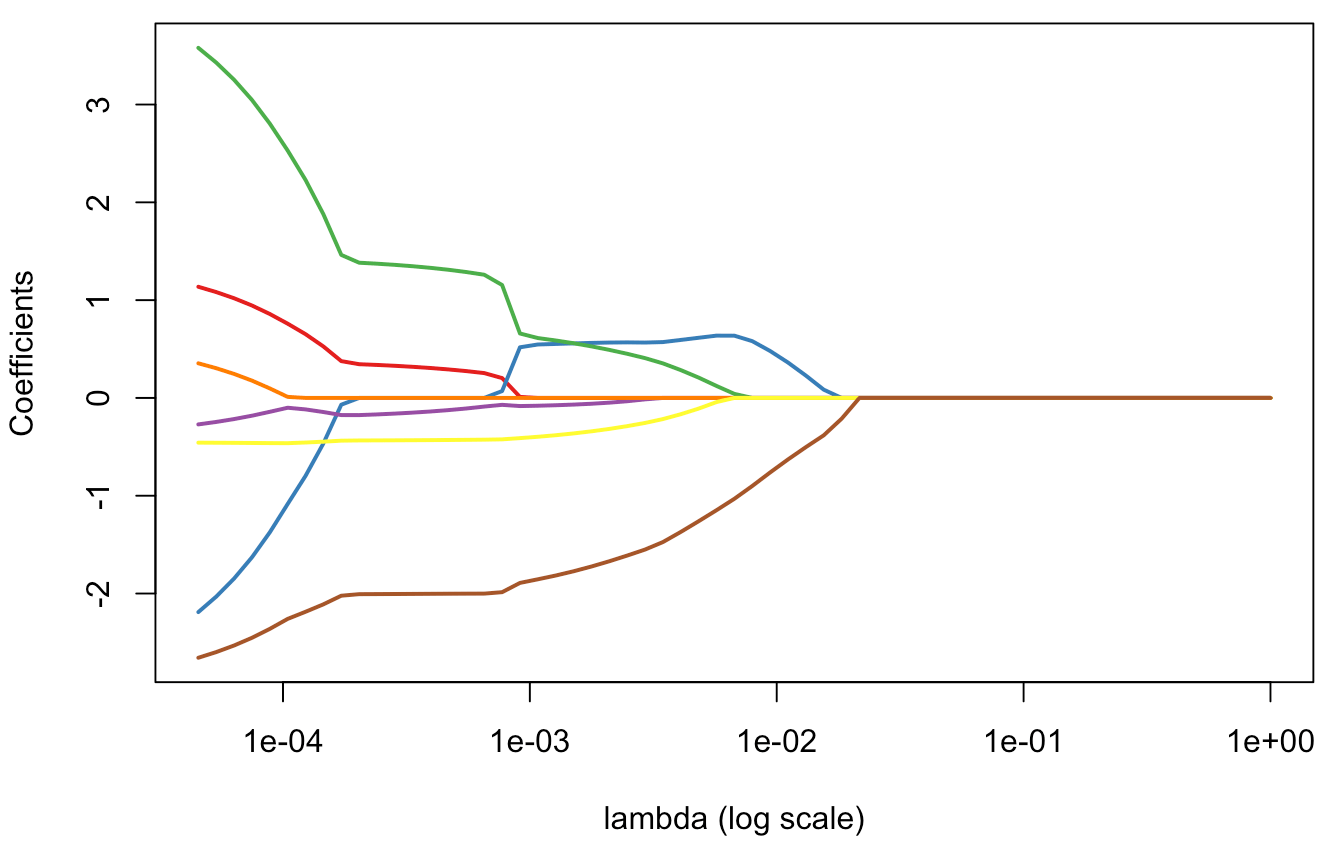It looks like what can get when calling glmnet… and here, we do have null components for some $\lambda$ large enough ! Really null… and that’s cool actually. ## Application on our second dataset Consider now the second dataset, with two covariates. The code to get lasso estimates is  1 2 3 4 5 6 7 8 9 10 11 12 13 14 15 16 17  df0 = df df0$y = as.numeric(df$y)-1 plot_lambda = function(lambda){ m = apply(df0,2,mean) s = apply(df0,2,sd) for(j in 1:2) df0[,j] <- (df0[,j]-m[j])/s[j] reg = glmnet(cbind(df0$x1,df0$x2), df0$y==1, alpha=1,lambda=lambda) u = seq(0,1,length=101) p = function(x,y){ xt = (x-m)/s yt = (y-m)/s predict(reg,newx=cbind(x1=xt,x2=yt),type="response")} v = outer(u,u,p) image(u,u,v,col=clr10,breaks=(0:10)/10) points(df$x1,df$x2,pch=19,cex=1.5,col="white") points(df$x1,df$x2,pch=c(1,19)[1+z],cex=1.5) contour(u,u,v,levels = .5,add=TRUE)}

Consider some small values, for [\lambda], so that we only have some sort of shrinkage of parameters,

 1 2 3 4 5  reg = glmnet(cbind(df0$x1,df0$x2), df0$y==1, alpha=1) par(mfrow=c(1,2)) plot(reg,xvar="lambda",col=c("blue","red"),lwd=2) abline(v=exp(-2.8)) plot_lambda(exp(-2.8))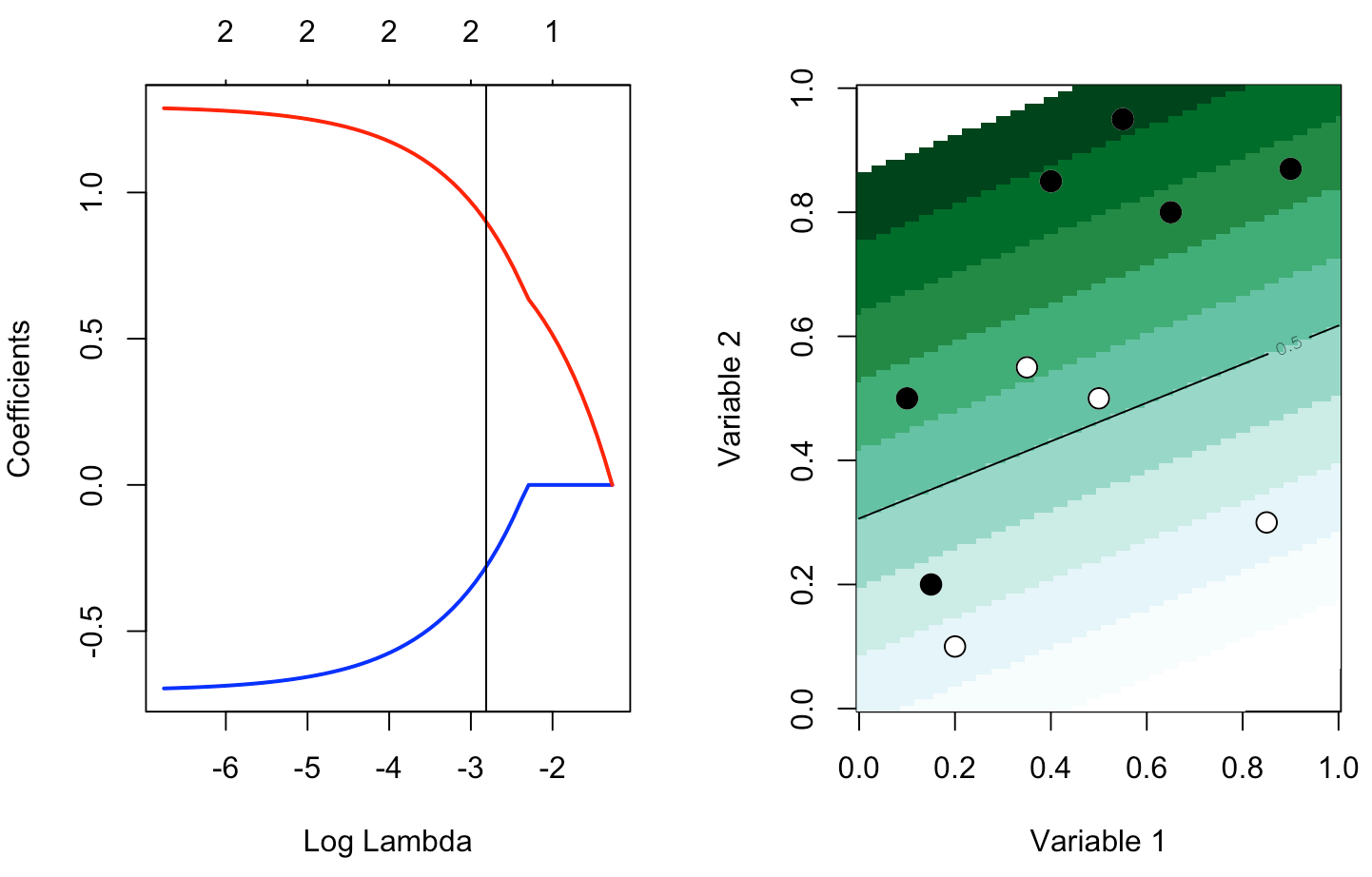But with a larger $\lambda$, there is variable selection: here $\widehat{\beta}_{1,\lambda}=0$  1 2 3 4 5  reg = glmnet(cbind(df0$x1,df0$x2), df0$y==1, alpha=1) par(mfrow=c(1,2)) plot(reg,xvar="lambda",col=c("blue","red"),lwd=2) abline(v=exp(-2.1)) plot_lambda(exp(-2.1))R-bloggers.com offers daily e-mail updates about R news and tutorials about learning R and many other topics. Click here if you're looking to post or find an R/data-science job.
Want to share your content on R-bloggers? click here if you have a blog, or here if you don't.